# To find: The values of KP, KM, MR, ML, MN and PR. Given: 12210203131.jpg bar(PR)||bar(KL) = 9, ln = 16, PM = 2(KP) KM=KP+PM=3KPQuestion
SimilarityTo find: The values of KP, KM, MR, ML, MN and PR.
Given: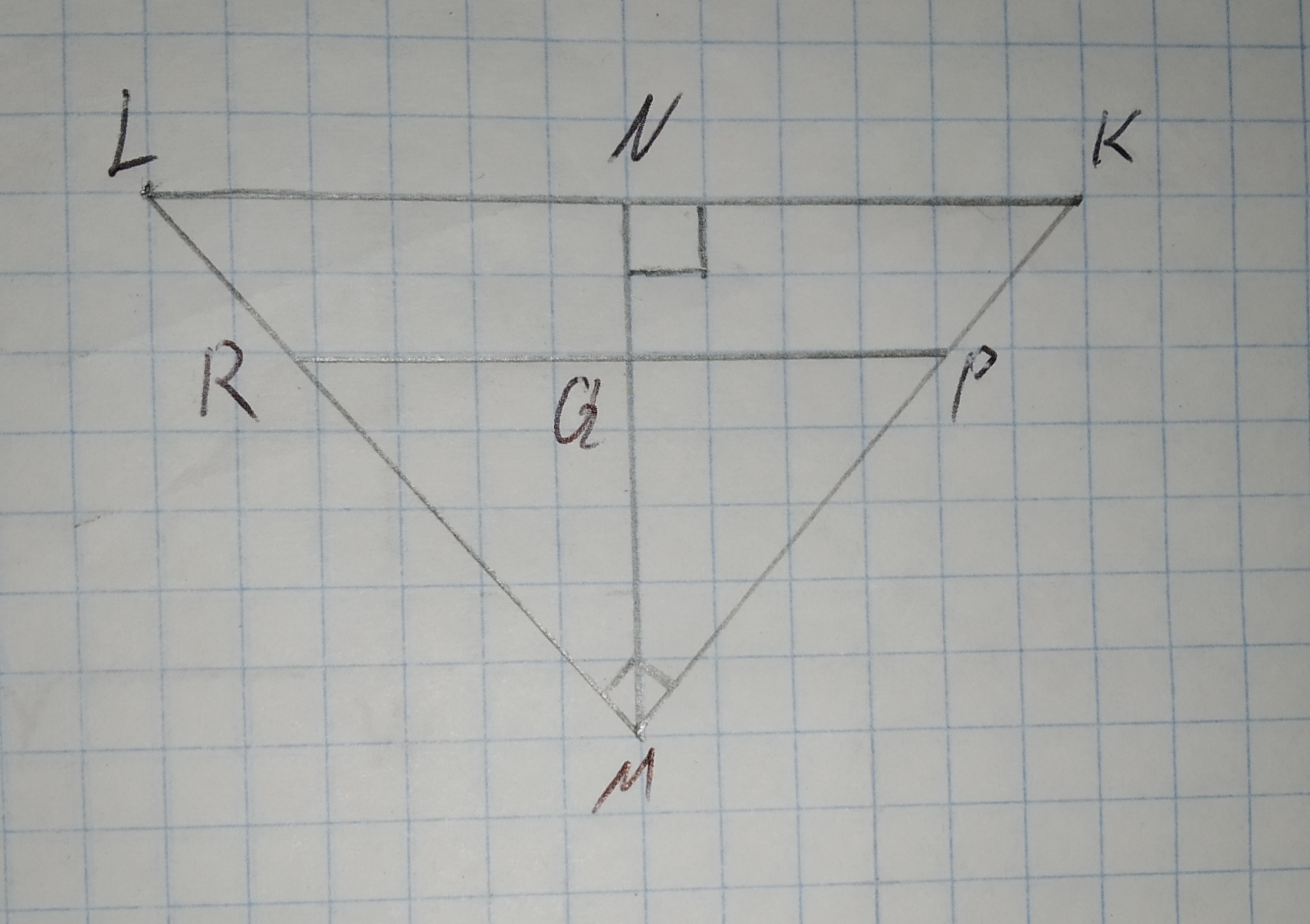$$\displaystyle\overline{{{P}{R}}}{\mid}{\mid}\overline{{{K}{L}}}={9},{\ln{=}}{16},{P}{M}={2}{\left({K}{P}\right)}$$
KM=KP+PM=3KP2021-01-11
Conclusion:
By A-A similarity, it can be proved that:
$$\displaystyle\triangle{L}{M}{K}\sim\triangle{M}{N}{K}$$
Similar triangles have corresponding sides as proportional.
$$\displaystyle\frac{{{M}{K}}}{{{L}{K}}}=\frac{{{N}{K}}}{{{M}{K}}}$$
$$\displaystyle\Rightarrow\frac{{{3}{K}{P}}}{{25}}=\frac{{9}}{{{3}{K}{P}}}$$
$$\displaystyle\Rightarrow{K}{P}={5}$$
$$\displaystyle\Rightarrow{K}{M}={3}{K}{P}={15}$$
By A-A similarity, it can be proved that:
$$\displaystyle\triangle{L}{M}{K}\sim\triangle{R}{M}{P}$$
Similar triangles have corresponding sides as proportional.
$$\displaystyle\frac{{{M}{K}}}{{{L}{K}}}=\frac{{{P}{M}}}{{{R}{P}}}$$
$$\displaystyle\Rightarrow\frac{{15}}{{25}}=\frac{{10}}{{{R}{P}}}$$
$$\displaystyle\Rightarrow{K}{P}={16}\frac{{2}}{{3}}$$
Using Pythagorean Theorem in right angled triangle $$\displaystyle\triangle{L}{M}{K}$$:
$$\displaystyle{H}{y}{p}{o}{t}{e}\nu{s}{e}^{{2}}={B}{a}{s}{e}^{{2}}+{P}{e}{r}{p}{e}{n}{d}{i}{c}\underline{{a}}{r}^{{2}}$$
$$\displaystyle={25}^{{2}}={15}^{{2}}+{L}{M}^{{2}}$$
$$\displaystyle\Rightarrow{L}{M}^{{2}}={400}$$
$$\displaystyle\Rightarrow{L}{M}={20}$$
In similar triangles: $$\displaystyle\triangle{L}{M}{K}\sim\triangle{M}{N}{K}$$
Ratio can be written as:
$$\displaystyle\frac{{{L}{M}}}{{{M}{K}}}=\frac{{{M}{N}}}{{{N}{K}}}$$
$$\displaystyle\Rightarrow\frac{{20}}{{15}}=\frac{{{M}{N}}}{{9}}$$
$$\displaystyle\Rightarrow{M}{N}={12}$$
$$\displaystyle{K}{P}={5},{K}{M}={15},{M}{R}={13}\frac{{1}}{{3}},{M}{L}={20},{M}{N}={12},{P}{R}={16}\frac{{2}}{{3}}$$

### Relevant QuestionsTo check: whether the additional information in the given option would be enough to prove the given similarity.
Given:
The given similarity is $$\displaystyle\triangle{A}{D}{C}\sim\triangle{B}{E}{C}$$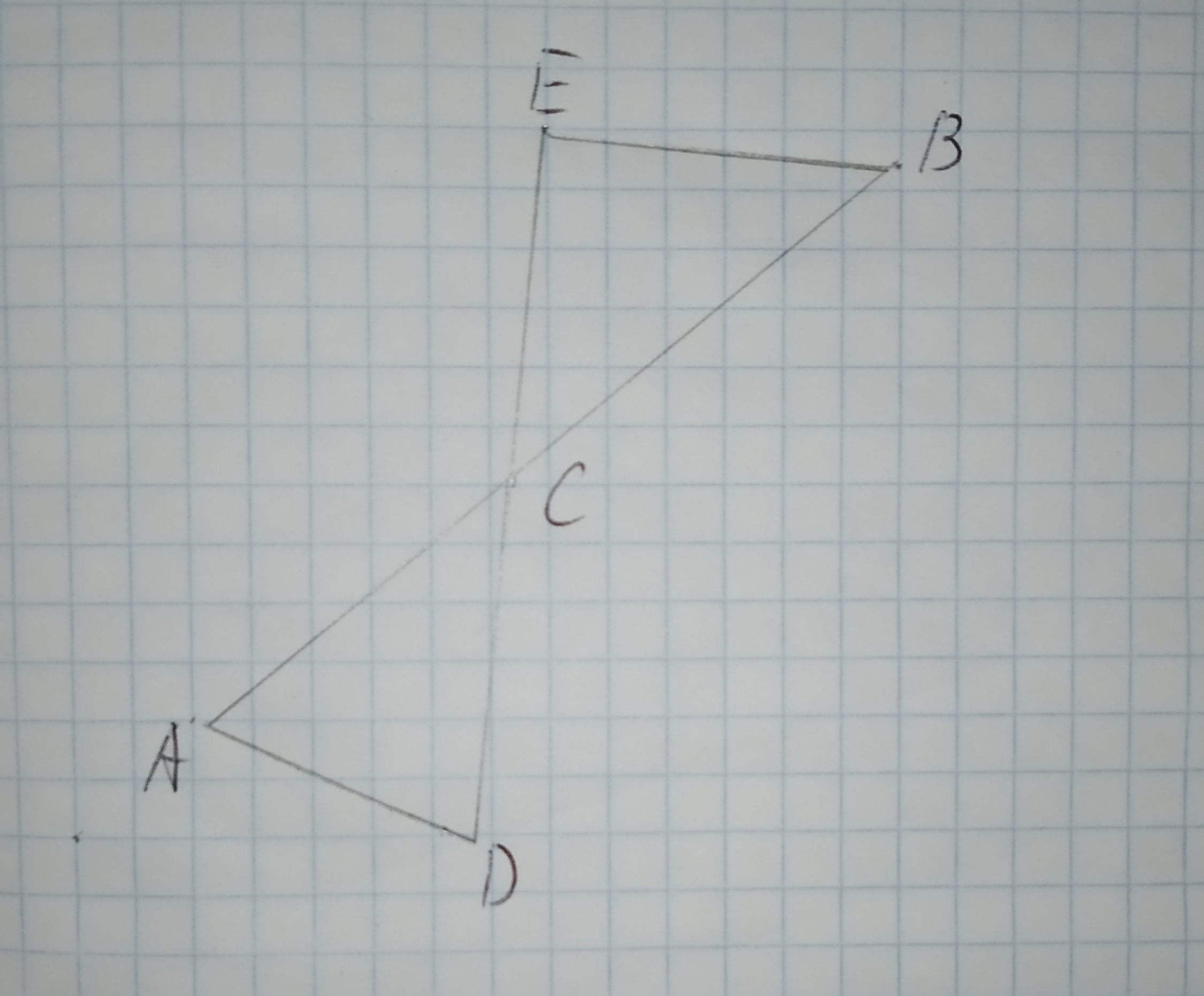The given options are:
A.$$\displaystyle\angle{D}{A}{C}{\quad\text{and}\quad}\angle{E}{C}{B}$$ are congruent.
B.$$\displaystyle\overline{{{A}{C}}}{\quad\text{and}\quad}\overline{{{B}{C}}}$$ are congruent.
C.$$\displaystyle\overline{{{A}{D}}}{\quad\text{and}\quad}\overline{{{E}{B}}}$$ are parallel.
D.$$\displaystyle\angle{C}{E}{B}$$ is a right triangle.Triangle MPT with $$\displaystyle\overline{{{N}{R}}}{\mid}{\mid}\overline{{{M}{T}}}$$ is shown below. The dimensions are in centimeters.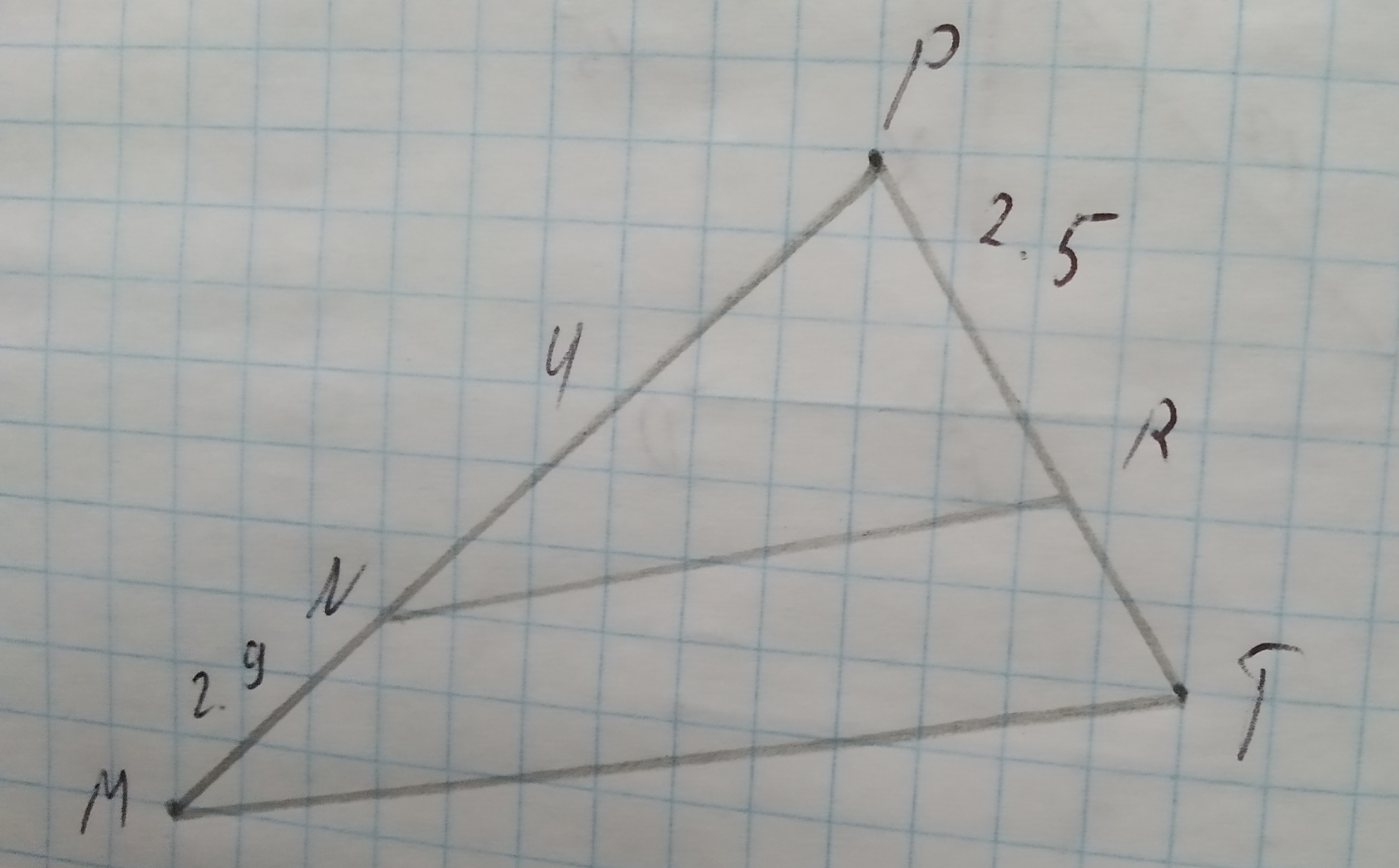Which measurement is closest to the length of $$\displaystyle\overline{{{R}{T}}}$$ in centimeters?
1)1.4
2)1.8
3)3.4
4)4.3To determine:To Find:the measure of side BB' in the figure shown such that VA' = 15, A'A=20 and A'B'=24
Given:
Figure is shown below.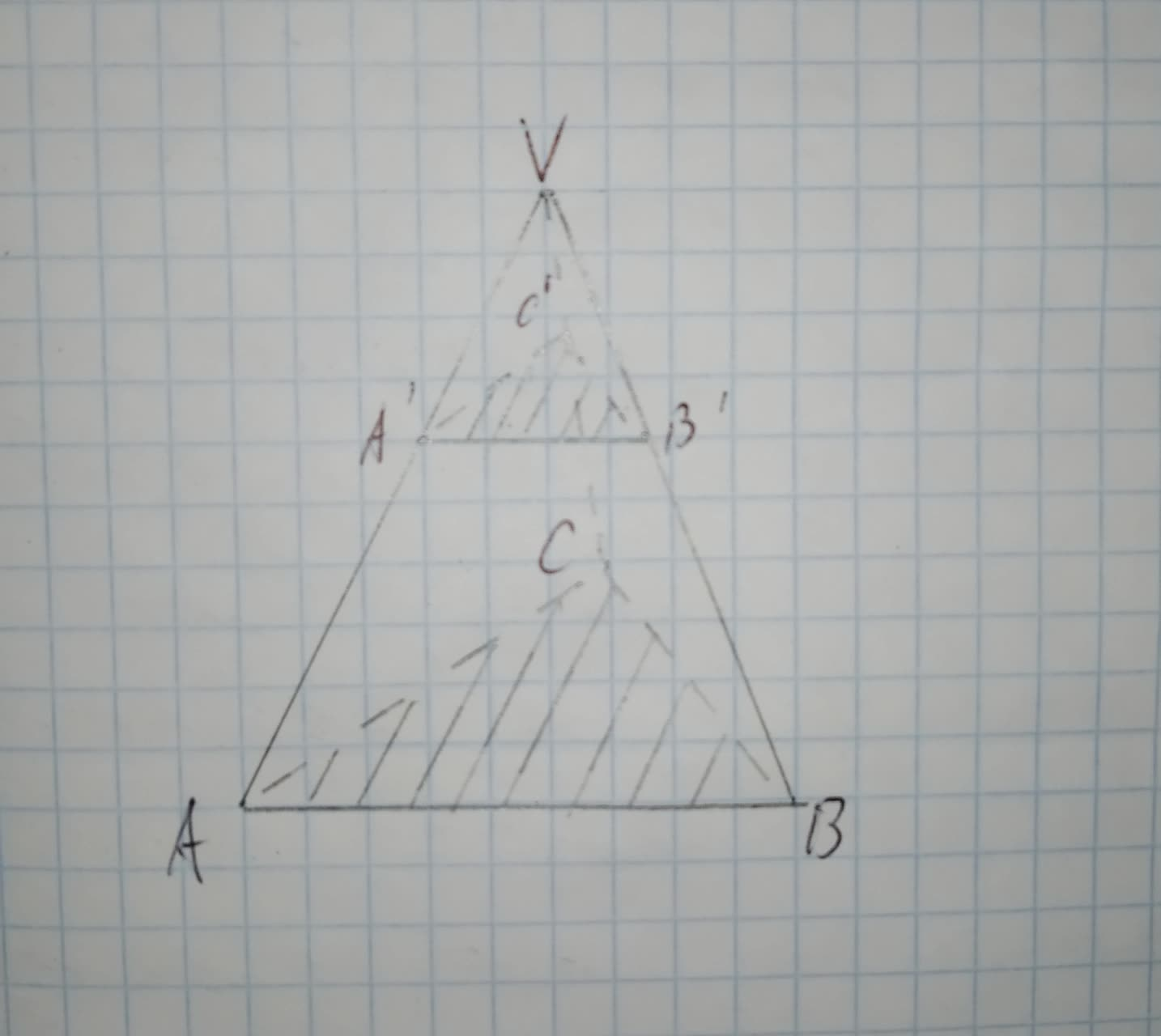To determine:To Find:the measure of side BB' in the figure shown such that VA' = 15, A'A=20 and VB=49
Given:
Figure is shown below.To determine:To Find:the measure of side VC in the figure shown such that VA' = 15, A'A=20 and VC'=18
Given:
Figure is shown below.To calculate:The ratio of line AB and AC.
Given information: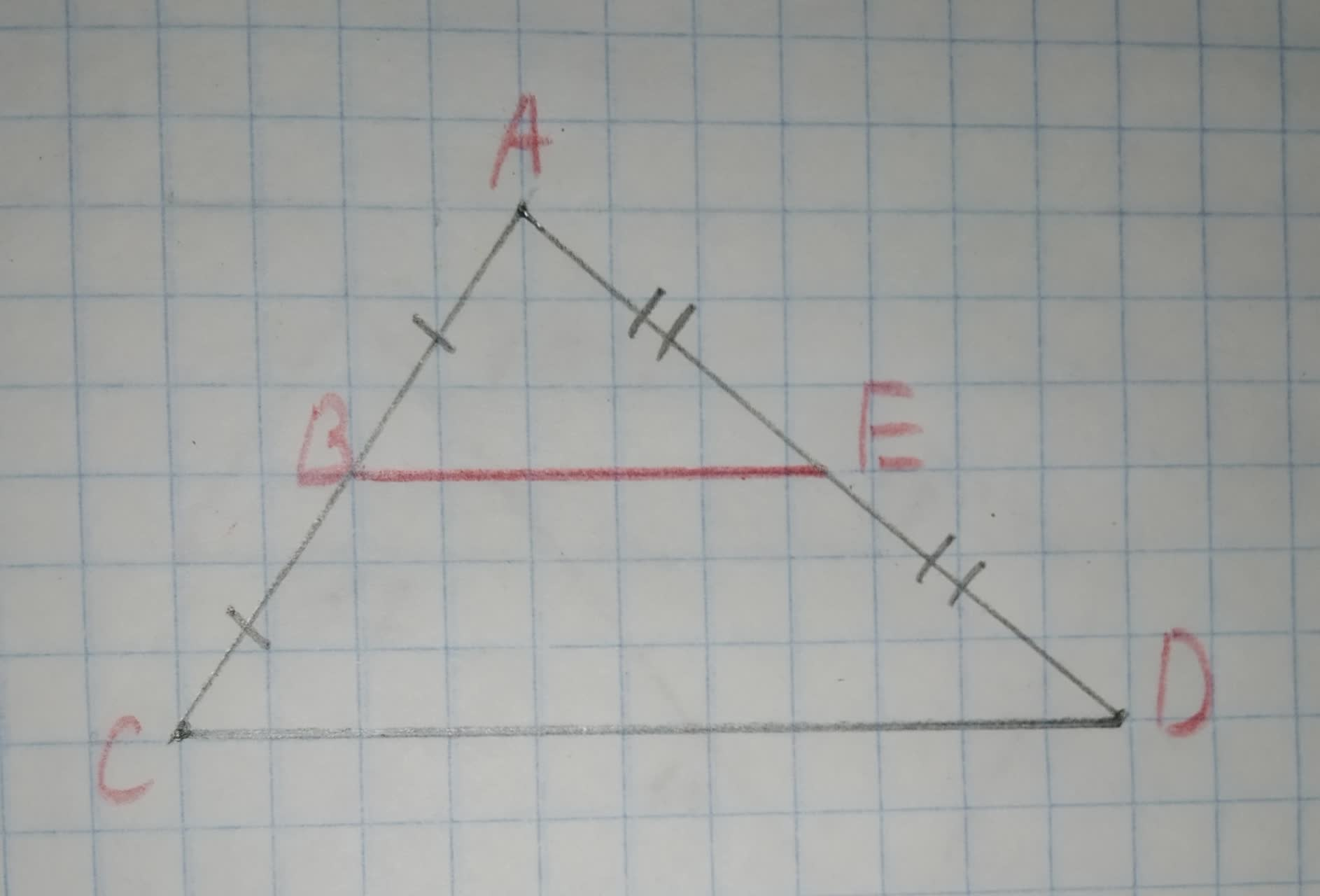To calculate:The ratio of line AB and BC.
Given information:
The following diagram is givenTo write: the name of the postulate, that justifies the given statement.
Given information:In $$\displaystyle\triangle{A}{V}{B}{\quad\text{and}\quad}\triangle{N}{V}{K},\angle{A}=\angle{N}={60}^{{\circ}}$$.
The given figure is as follows: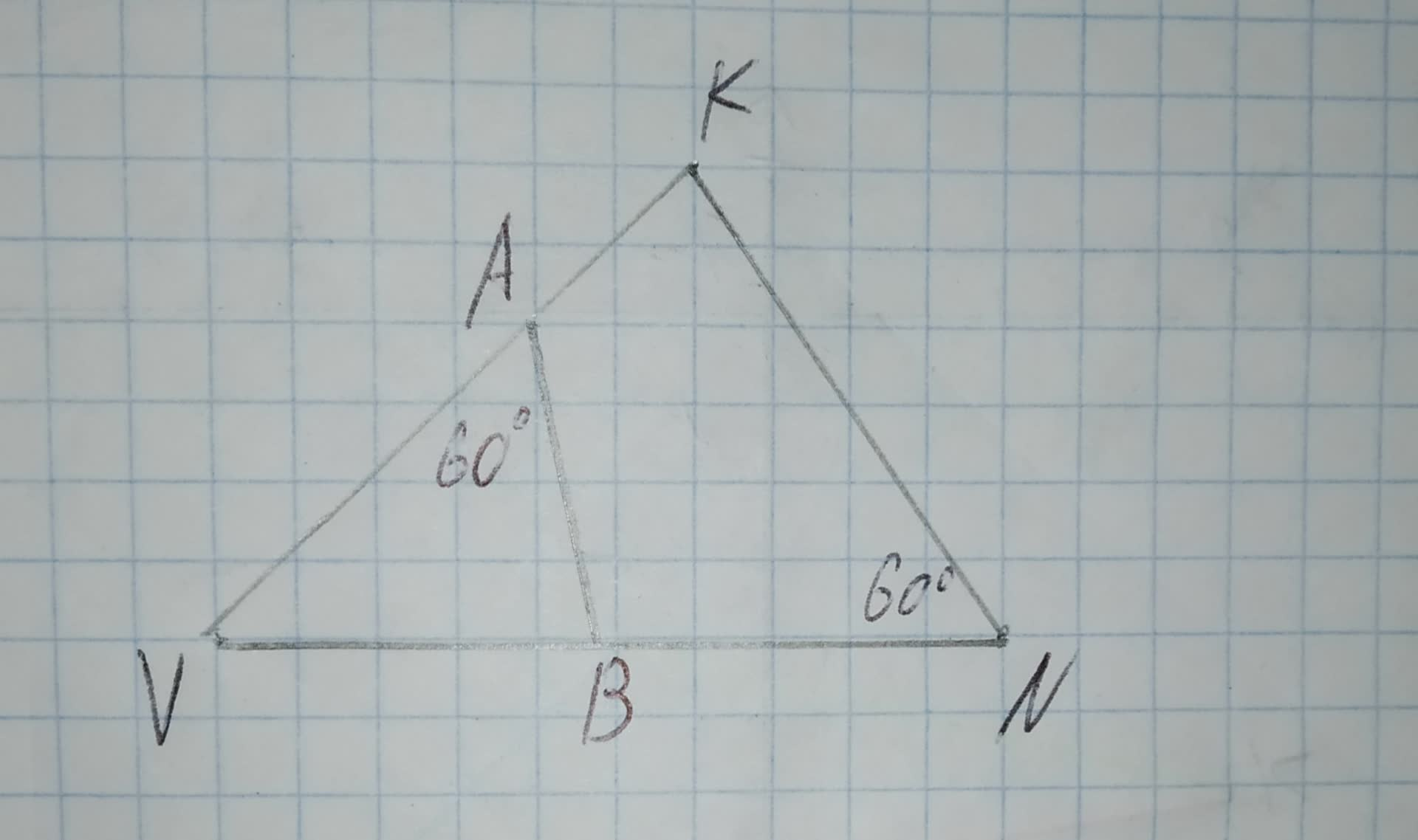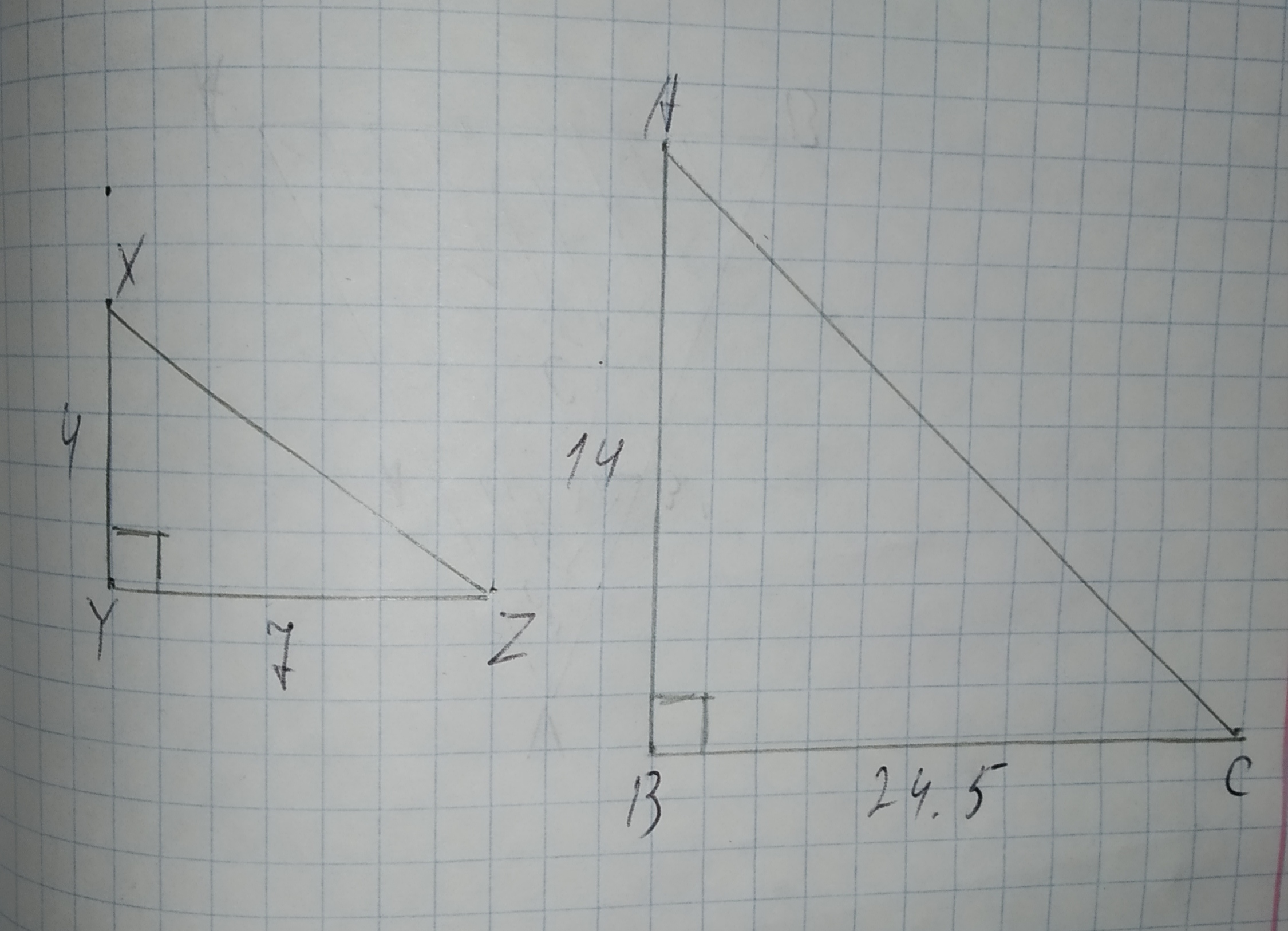To prove: The congruency of $$\displaystyle\triangle{R}{K}{M}\stackrel{\sim}{=}\triangle{O}{M}{K}$$.
$$\displaystyle\angle{M}{R}{K}=\angle{K}{O}{M}={90}^{{\circ}}$$
$$\displaystyle\angle{R}{K}{M}=\angle{O}{M}{K}$$